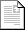ASEE Zone 2 Conference 2017

Proceedings »

Betting your Grade: Using Introductory Statistics to Teach Data-Driven Decision Making

 Final Paperpdf140KB

This paper describes a technique for enhancing student motivation and providing meaningful context for introductory statistics. Additionally, students learn how to make – and the importance of – data-based decisions. Sophomore students in Grove City College’s experimental methods lab course were unable to perform or understand basic statistical data analyses such as confidence intervals, regression and tests of hypotheses. Basic instruction using Matlab’s statistical toolbox allowed some students to perform the analyses by rote, but the understanding was poor, and many students continued to struggle. In 2014, the structure of the course was changed, reducing the number of experiments and increasing the time devoted to data analysis by spending two weeks on each lab. During the first week, students conduct the experiment and record data, followed by an introduction to the statistics principles required to answer the lab questions. The following week, after a more detailed explanation, students are required to demonstrate an understanding of the statistical method by making predictions based on their own measured data. For example, in an experiment involving linear regression, students use their data from the previous week and a regression model to predict the outcome of three new trials for which the instructor provided values of the independent variable. The students then experimentally measure the outcomes. Their grade for the demonstration depend on the width of their prediction intervals and the fraction of trials within their predicted intervals. This provides very high motivation for learning the statistical method, and also set an example for how engineers make decisions based on data. Application of statistics to their end-of-semester student-design lab assignment show that students better understand statistical methods and their applications. Other metrics, such as quiz scores and course evaluations, did not show clear improvement, possibly due to increased rigor on the quizzes.

Author(s):

Mark Archibald
Mechanical Engineering
Grove City College
United States